4WX Internet Search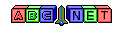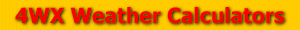# Altimeter Setting

From the user, a station pressure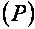and a station elevation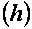are given. To calculate altimeter setting, the station pressure must be in units of millibars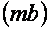. To see how to convert station pressure to millibars see the link below:

pressureConversion.php

Also to calculate the altimeter setting, the station elevation must be in units of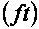. To convert meters to feet, see the equation below: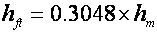Then, the altimeter setting can be calculated using the formula below:Then, the answer can be converted to different pressure units. See the link above to see how to do this.

Back to 4WX Weather Calculators index page

4WX Calculators Credit and disclaimer